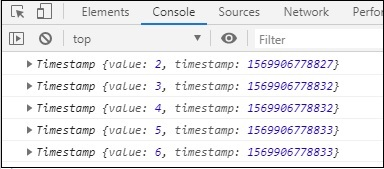# RxJS - Utility Operator timestamp

Returns the timestamp along with the value emitted from source Observable which tells about the time when the value was emitted.

## Syntax

```timestamp(): Observable
```

## Return value

Returns the timestamp along with the value emitted from source Observable which tells about the time when the value was emitted.

## Example

```import { of } from 'rxjs';
import { filter, timestamp } from 'rxjs/operators';

let list1 = of(2, 3, 4, 5, 6);
let final_val = list1.pipe(timestamp());
final_val.subscribe(x => console.log(x));
```

## Output